Скачать презентацию New quantum lower bound method with applications to

• Количество слайдов: 53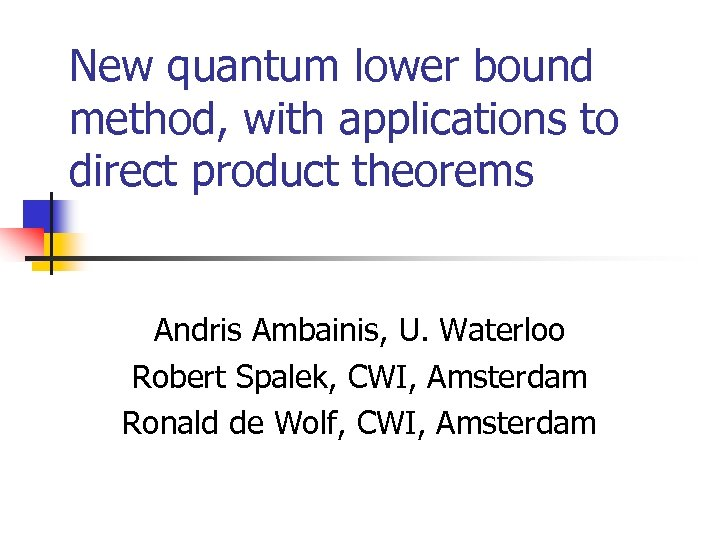New quantum lower bound method, with applications to direct product theorems Andris Ambainis, U. Waterloo Robert Spalek, CWI, Amsterdam Ronald de Wolf, CWI, Amsterdam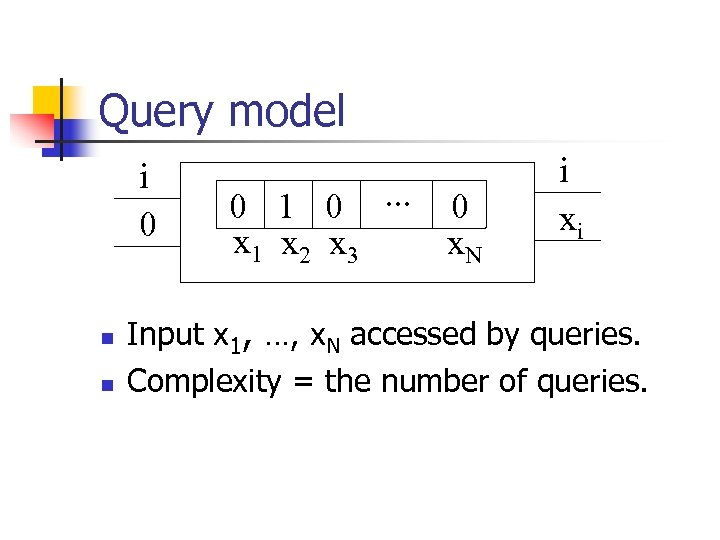Query model i 0 n n 0 1 0. . . 0 x 1 x 2 x 3 x. N i xi Input x 1, …, x. N accessed by queries. Complexity = the number of queries.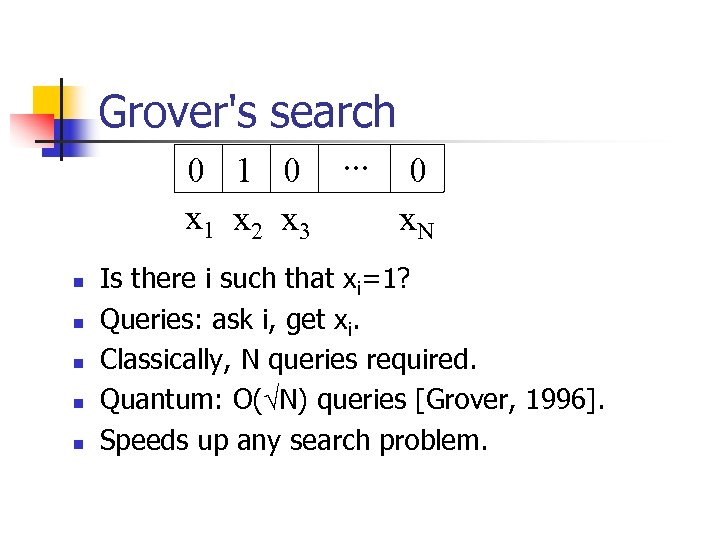Grover's search 0 1 0. . . 0 x 1 x 2 x 3 x. N n n n Is there i such that xi=1? Queries: ask i, get xi. Classically, N queries required. Quantum: O( N) queries [Grover, 1996]. Speeds up any search problem.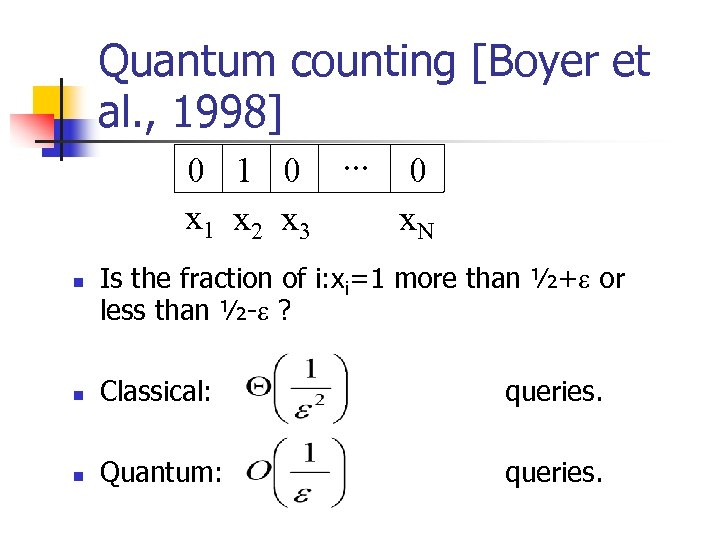Quantum counting [Boyer et al. , 1998] 0 1 0. . . 0 x 1 x 2 x 3 x. N n Is the fraction of i: xi=1 more than ½+ or less than ½- ? n Classical: queries. n Quantum: queries.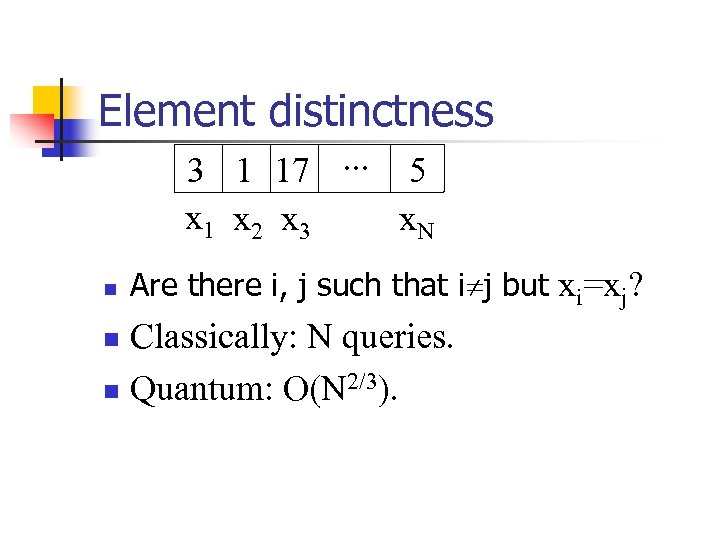Element distinctness 3 1 17. . . 5 x 1 x 2 x 3 x. N n Are there i, j such that i j but xi=xj? Classically: N queries. n Quantum: O(N 2/3). n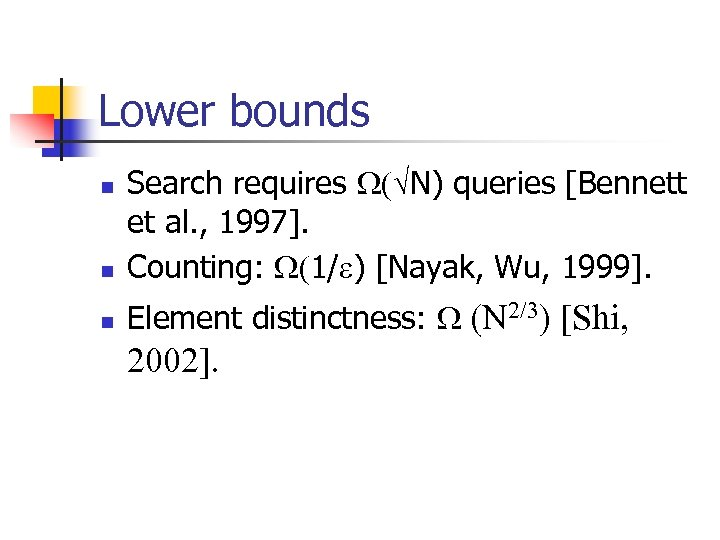Lower bounds n Search requires N) queries [Bennett et al. , 1997]. Counting: 1/ ) [Nayak, Wu, 1999]. n Element distinctness: (N 2/3) [Shi, n 2002].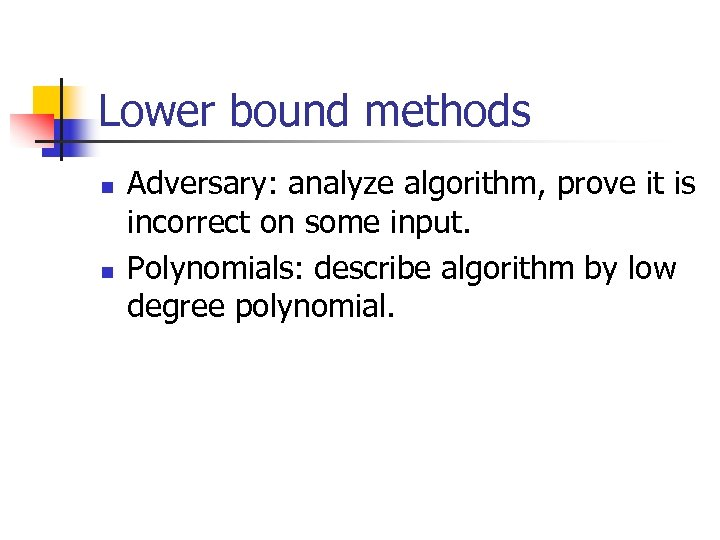Lower bound methods n n Adversary: analyze algorithm, prove it is incorrect on some input. Polynomials: describe algorithm by low degree polynomial.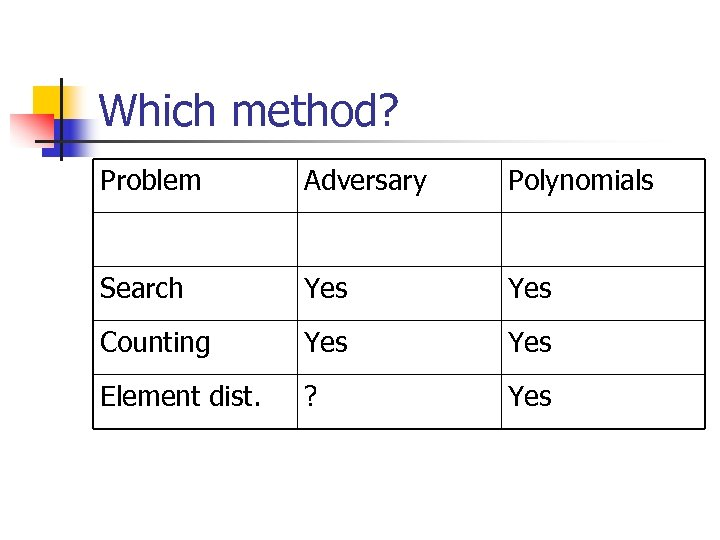Which method? Problem Adversary Polynomials Search Yes Counting Yes Element dist. ? Yes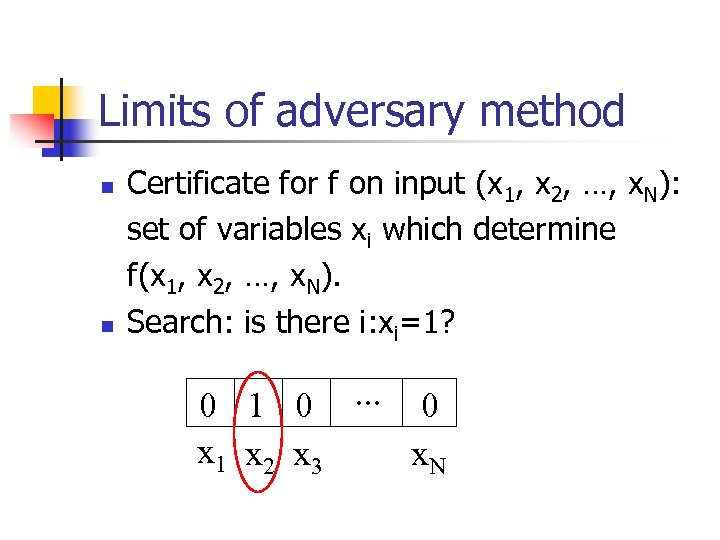Limits of adversary method n n Certificate for f on input (x 1, x 2, …, x. N): set of variables xi which determine f(x 1, x 2, …, x. N). Search: is there i: xi=1? 0 1 0. . . 0 x 1 x 2 x 3 x. N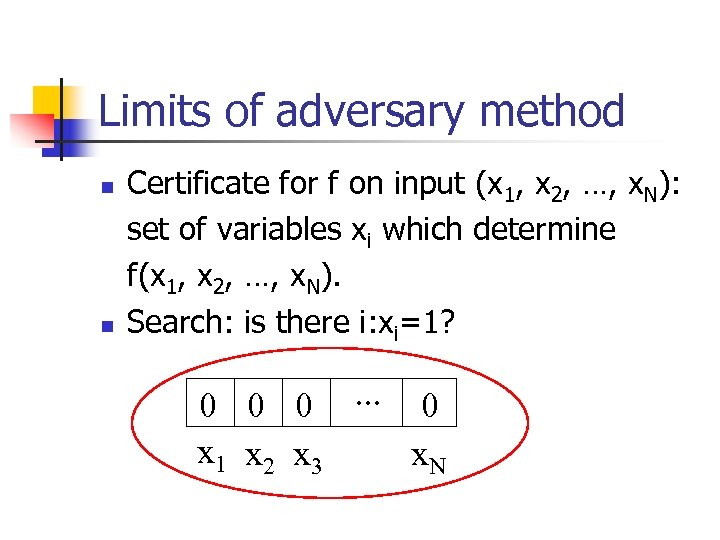Limits of adversary method n n Certificate for f on input (x 1, x 2, …, x. N): set of variables xi which determine f(x 1, x 2, …, x. N). Search: is there i: xi=1? 0 0 0. . . 0 x 1 x 2 x 3 x. N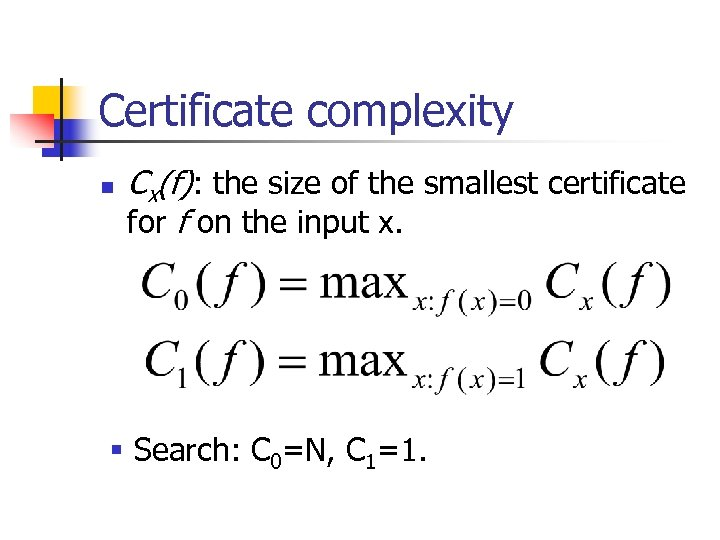Certificate complexity n Cx(f): the size of the smallest certificate for f on the input x. § Search: C 0=N, C 1=1.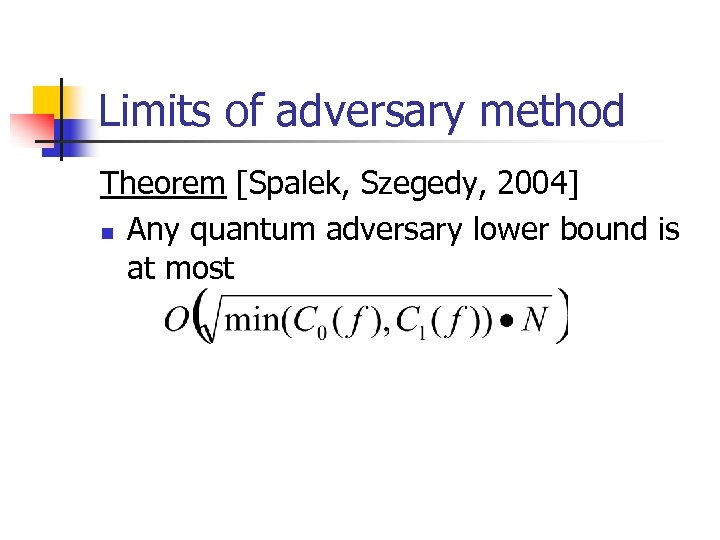Limits of adversary method Theorem [Spalek, Szegedy, 2004] n Any quantum adversary lower bound is at most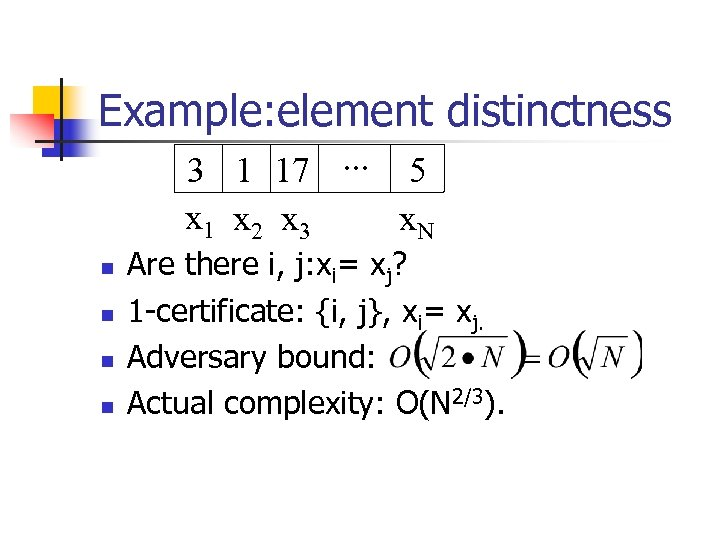Example: element distinctness 3 1 17. . . 5 x 1 x 2 x 3 x. N n n Are there i, j: xi= xj? 1 -certificate: {i, j}, xi= xj. Adversary bound: Actual complexity: O(N 2/3).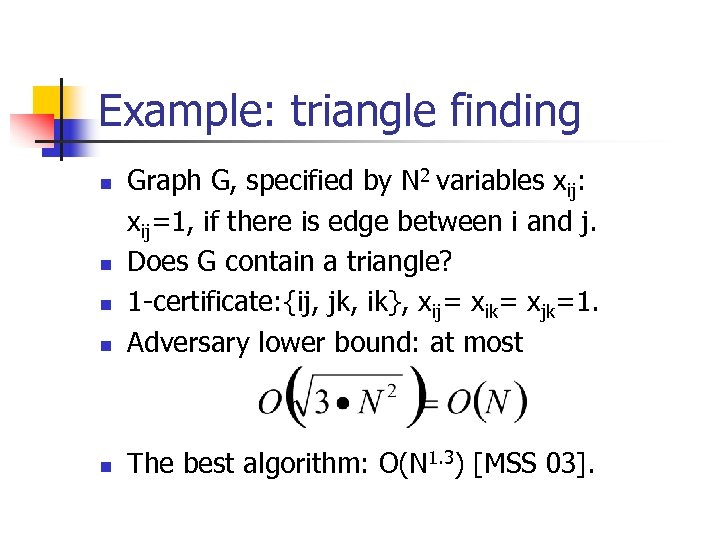Example: triangle finding n Graph G, specified by N 2 variables xij: xij=1, if there is edge between i and j. Does G contain a triangle? 1 -certificate: {ij, jk, ik}, xij= xik= xjk=1. Adversary lower bound: at most n The best algorithm: O(N 1. 3) [MSS 03]. n n n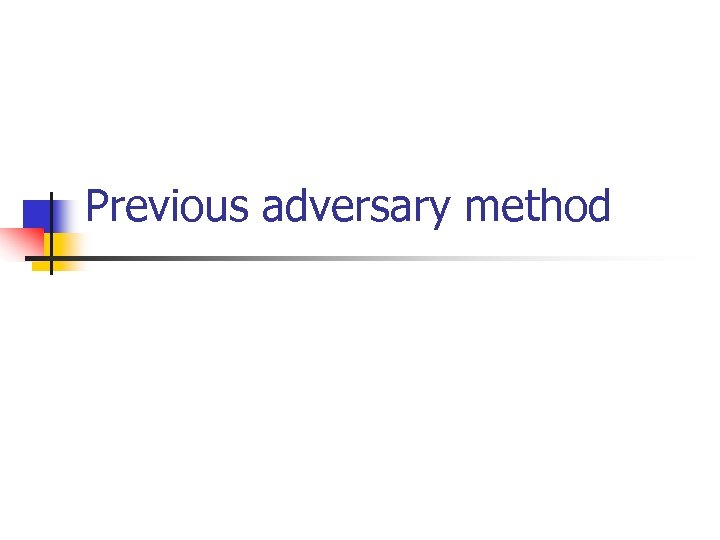Previous adversary method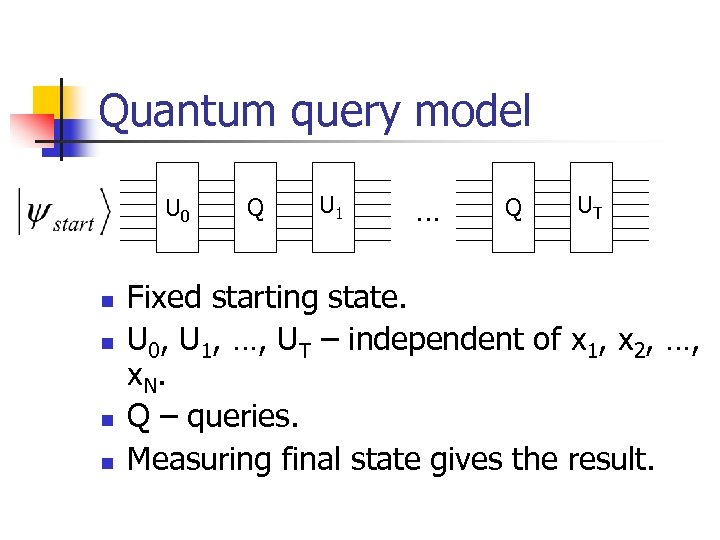Quantum query model U 0 n n Q U 1 … Q UT Fixed starting state. U 0, U 1, …, UT – independent of x 1, x 2, …, x. N. Q – queries. Measuring final state gives the result.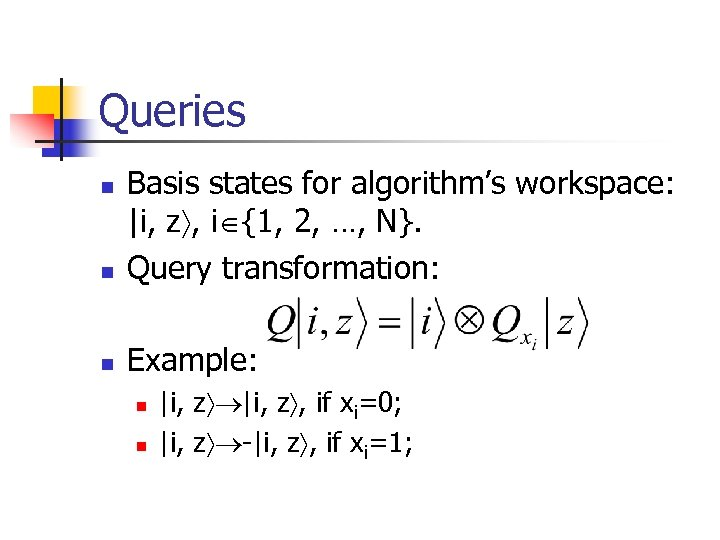Queries n Basis states for algorithm’s workspace: |i, z , i {1, 2, …, N}. Query transformation: n Example: n n n |i, z , if xi=0; |i, z -|i, z , if xi=1;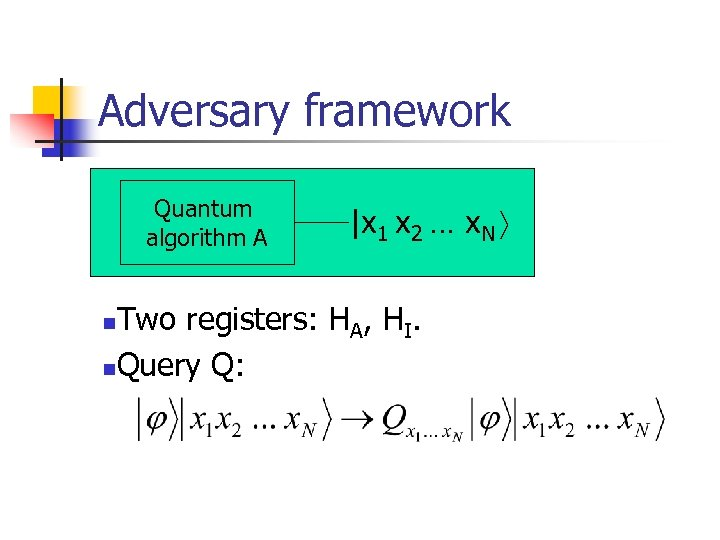Adversary framework Quantum algorithm A |x 1 x 2 … x. N Two registers: HA, HI. n. Query Q: n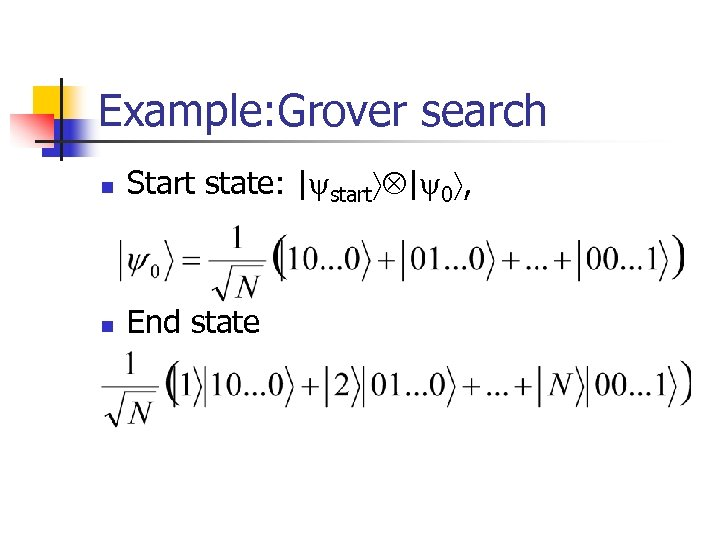Example: Grover search n Start state: | start | 0 , n End state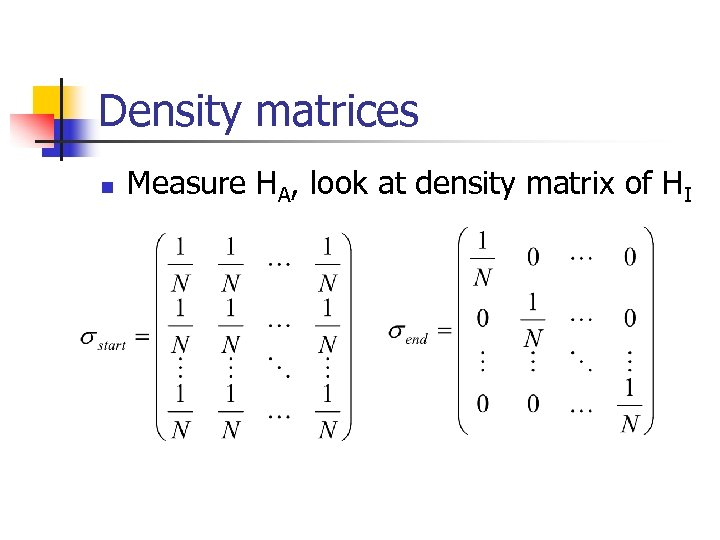Density matrices n Measure HA, look at density matrix of HI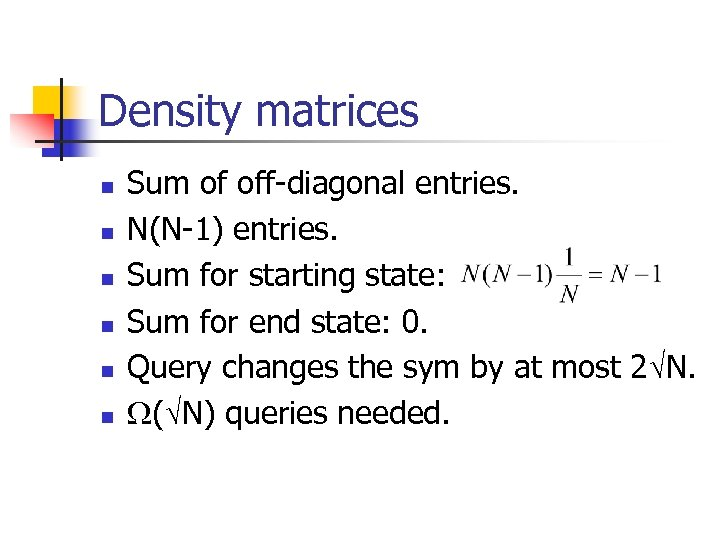Density matrices n n n Sum of off-diagonal entries. N(N-1) entries. Sum for starting state: Sum for end state: 0. Query changes the sym by at most 2 N. ( N) queries needed.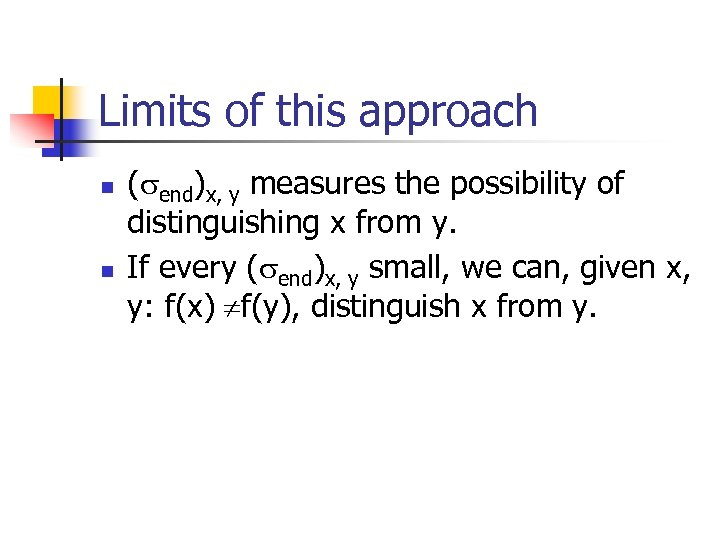Limits of this approach n n ( end)x, y measures the possibility of distinguishing x from y. If every ( end)x, y small, we can, given x, y: f(x) f(y), distinguish x from y.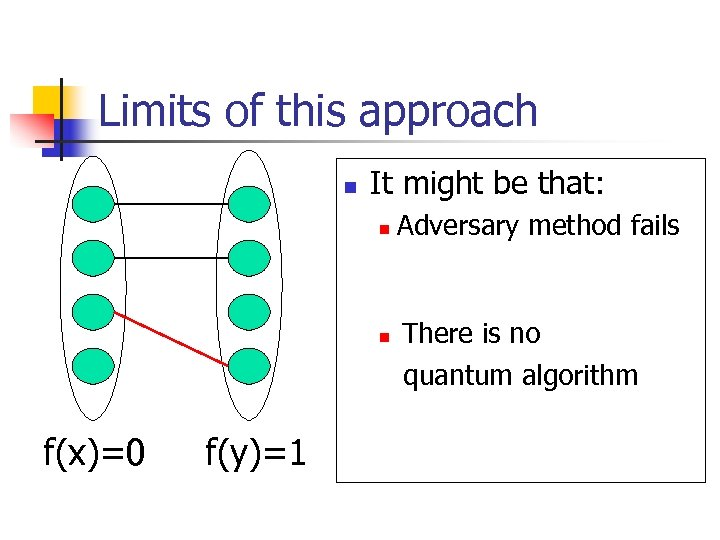Limits of this approach n It might be that: Every x can be Adversary method fails distinguished from every y; n There is no measurement that quantum algorithm distinguishes all x from all y. n f(x)=0 f(y)=1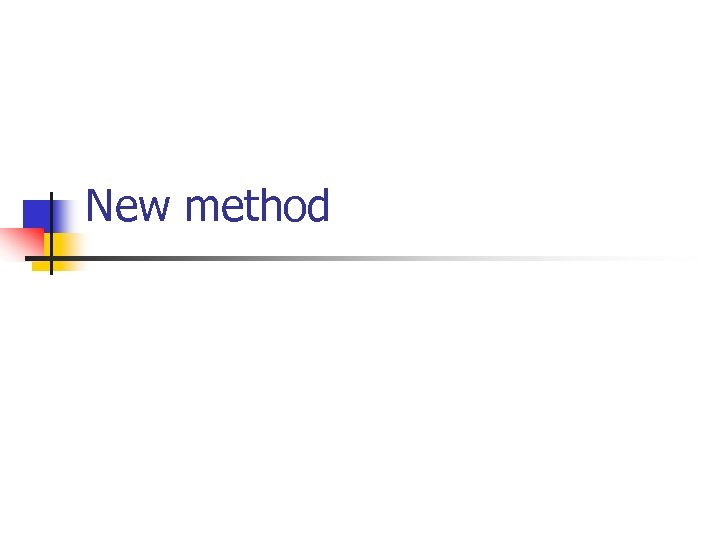New method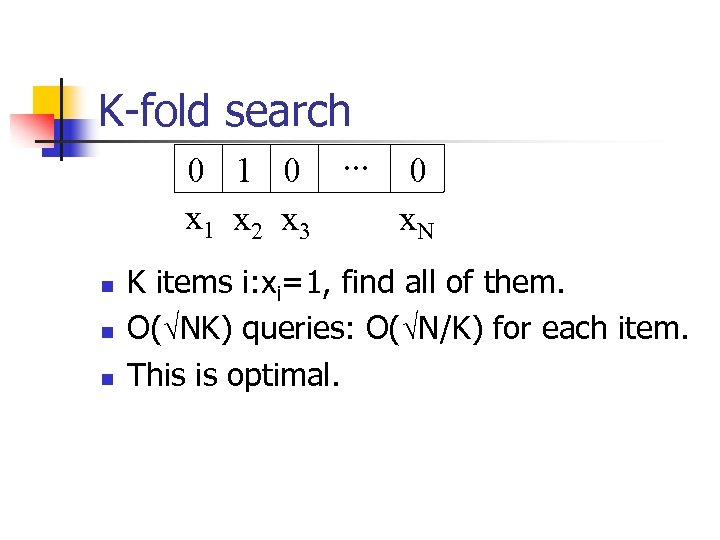K-fold search 0 1 0. . . 0 x 1 x 2 x 3 x. N n n n K items i: xi=1, find all of them. O( NK) queries: O( N/K) for each item. This is optimal.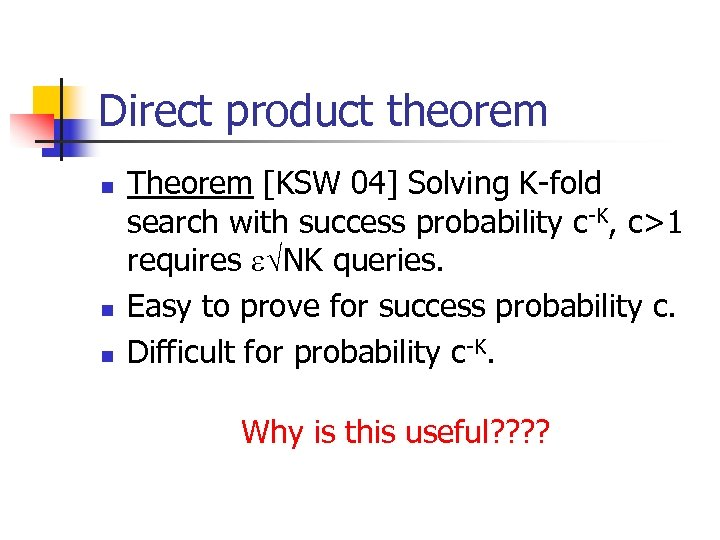Direct product theorem n n n Theorem [KSW 04] Solving K-fold search with success probability c-K, c>1 requires NK queries. Easy to prove for success probability c. Difficult for probability c-K. Why is this useful? ?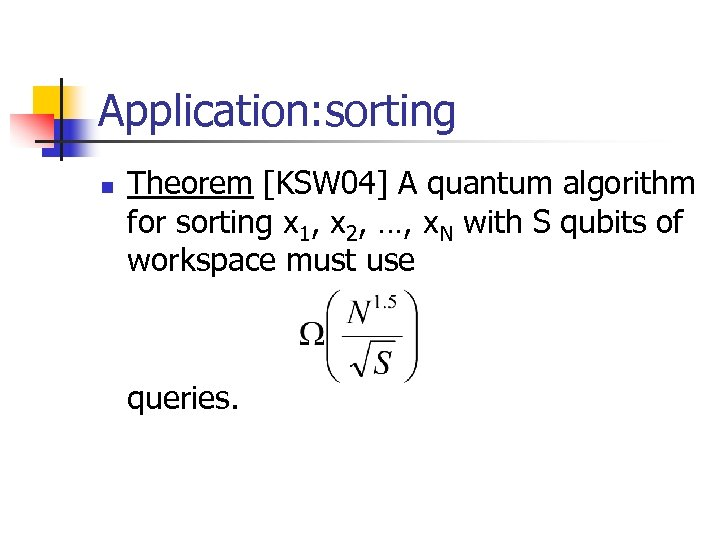Application: sorting n Theorem [KSW 04] A quantum algorithm for sorting x 1, x 2, …, x. N with S qubits of workspace must use queries.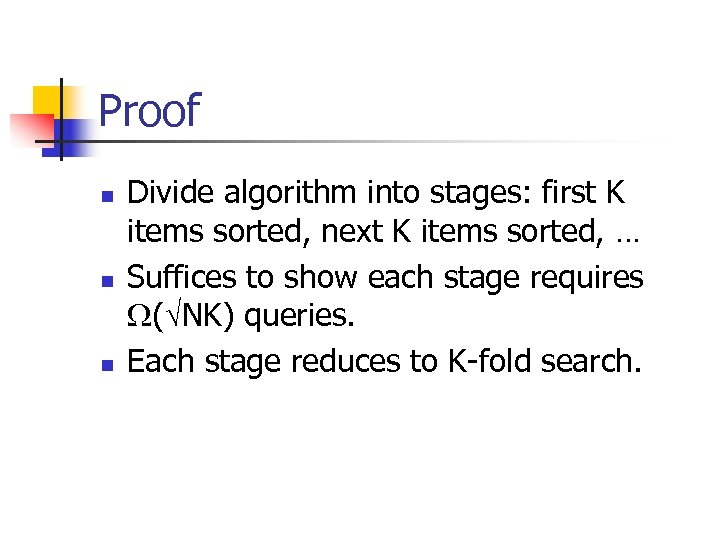Proof n n n Divide algorithm into stages: first K items sorted, next K items sorted, … Suffices to show each stage requires ( NK) queries. Each stage reduces to K-fold search.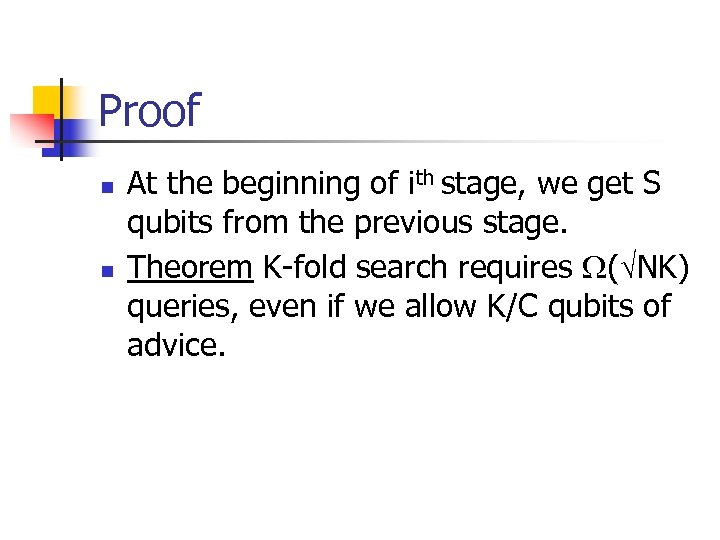Proof n n At the beginning of ith stage, we get S qubits from the previous stage. Theorem K-fold search requires ( NK) queries, even if we allow K/C qubits of advice.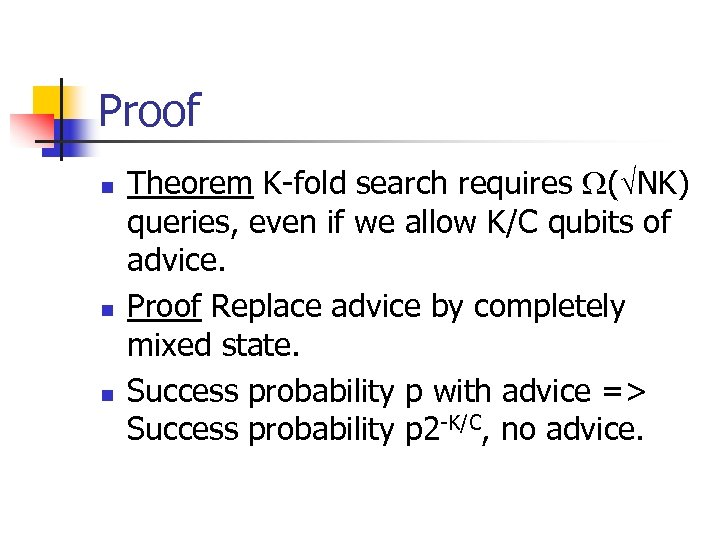Proof n n n Theorem K-fold search requires ( NK) queries, even if we allow K/C qubits of advice. Proof Replace advice by completely mixed state. Success probability p with advice => Success probability p 2 -K/C, no advice.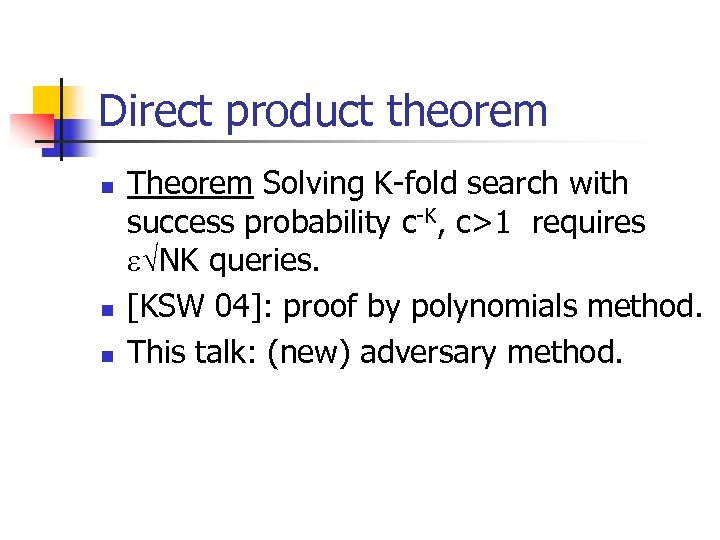Direct product theorem n n n Theorem Solving K-fold search with success probability c-K, c>1 requires NK queries. [KSW 04]: proof by polynomials method. This talk: (new) adversary method.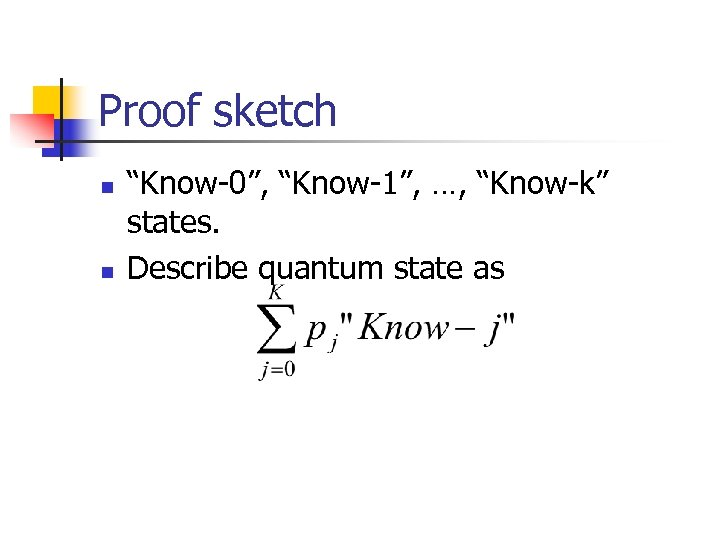Proof sketch n n “Know-0”, “Know-1”, …, “Know-k” states. Describe quantum state as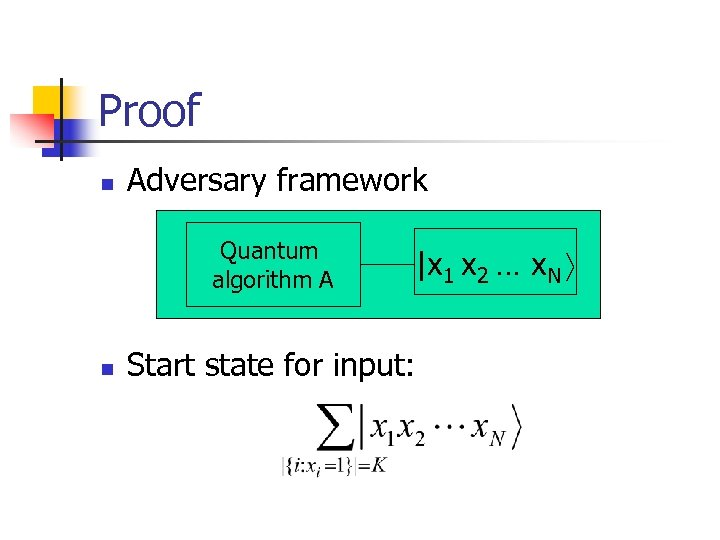Proof n Adversary framework Quantum algorithm A n |x 1 x 2 … x. N Start state for input: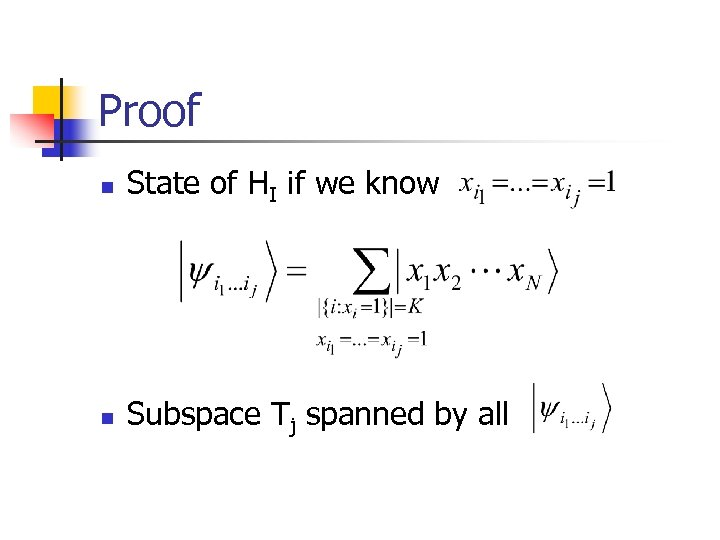Proof n State of HI if we know n Subspace Tj spanned by all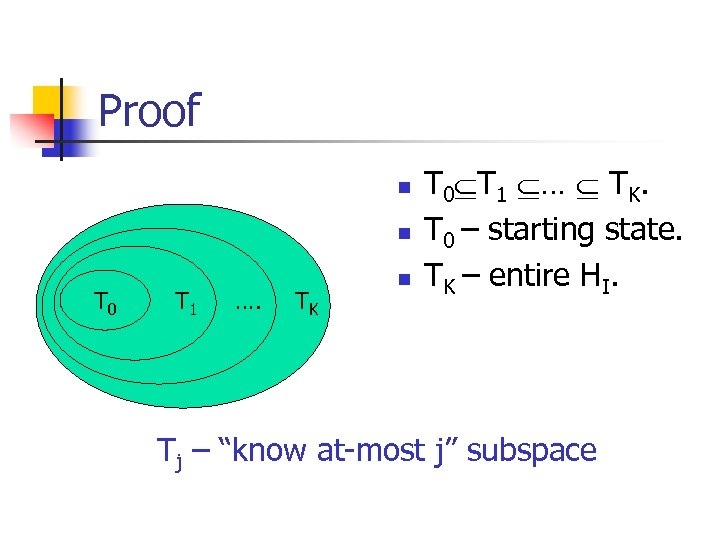Proof n n T 0 T 1 …. TK n T 0 T 1 … TK. T 0 – starting state. TK – entire HI. Tj – “know at-most j” subspace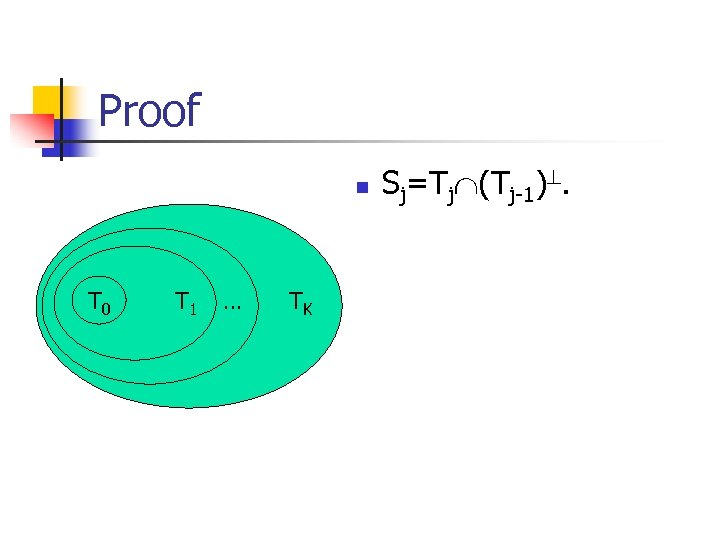Proof n T 0 T 1 … TK Sj=Tj (Tj-1).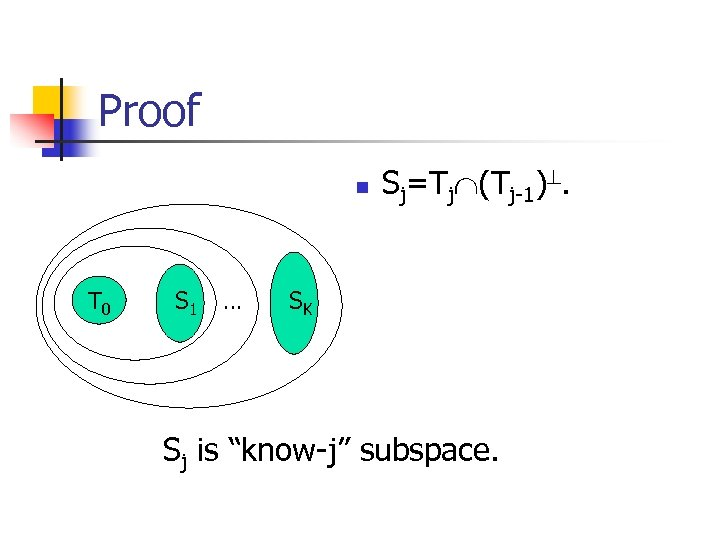Proof n T 0 S 1 … Sj=Tj (Tj-1). SK Sj is “know-j” subspace.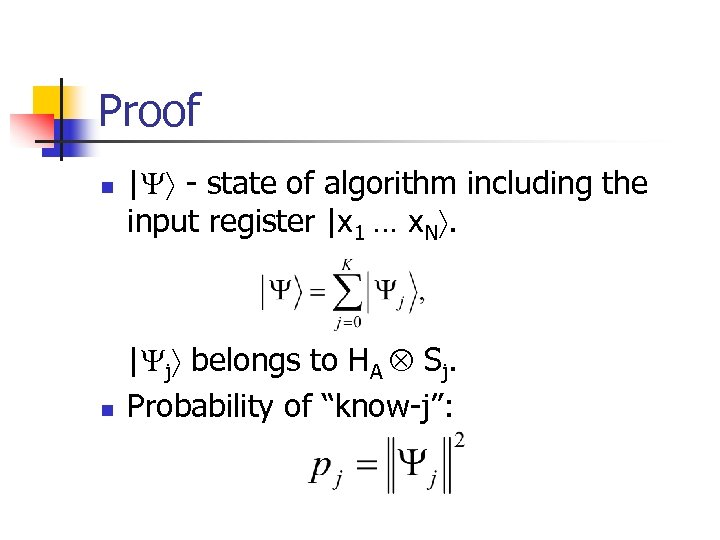Proof n n | - state of algorithm including the input register |x 1 … x. N. | j belongs to HA Sj. Probability of “know-j”: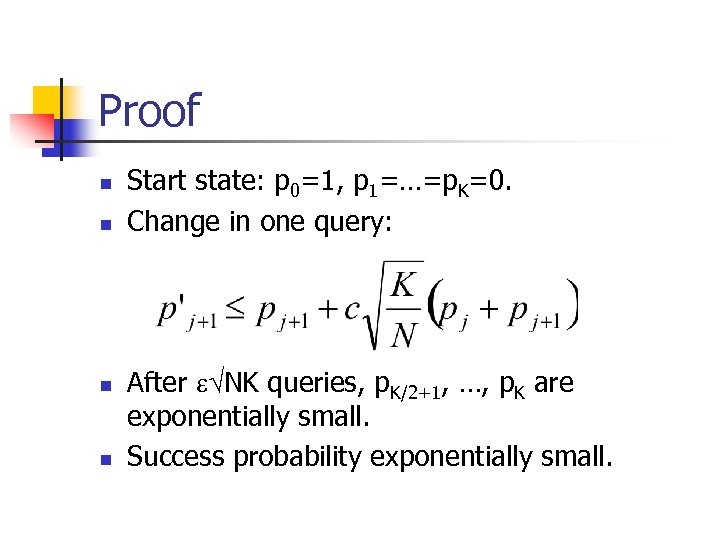Proof n n Start state: p 0=1, p 1=…=p. K=0. Change in one query: After NK queries, p. K/2+1, …, p. K are exponentially small. Success probability exponentially small.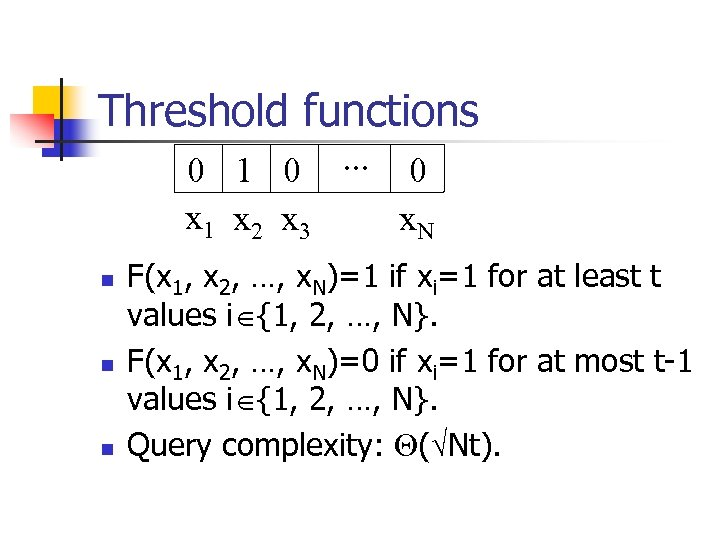Threshold functions 0 1 0. . . 0 x 1 x 2 x 3 x. N n n n F(x 1, x 2, …, x. N)=1 if xi=1 for at least t values i {1, 2, …, N}. F(x 1, x 2, …, x. N)=0 if xi=1 for at most t-1 values i {1, 2, …, N}. Query complexity: ( Nt).Threshold functions 0 1 0. . . 0 x 1 x 2 x 3 x. N n n n F(x 1, x 2, …, x. N)=1 if xi=1 for at least t values i {1, 2, …, N}. F(x 1, x 2, …, x. N)=0 if xi=1 for at most t-1 values i {1, 2, …, N}. Query complexity: ( Nt).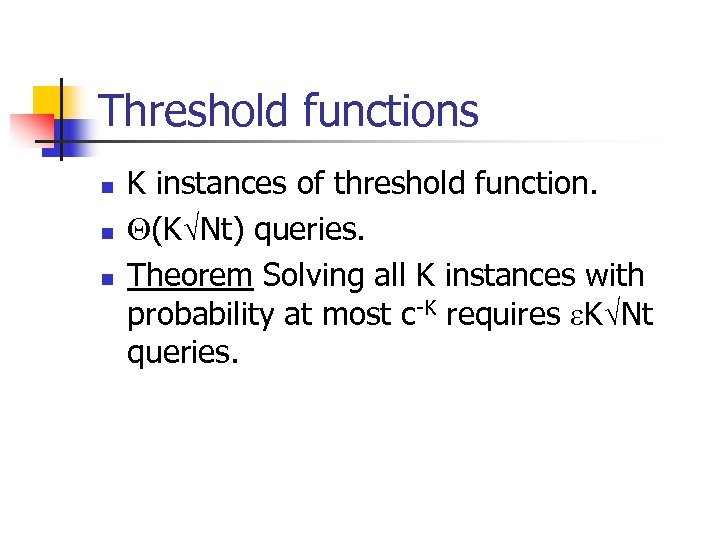Threshold functions n n n K instances of threshold function. (K Nt) queries. Theorem Solving all K instances with probability at most c-K requires K Nt queries.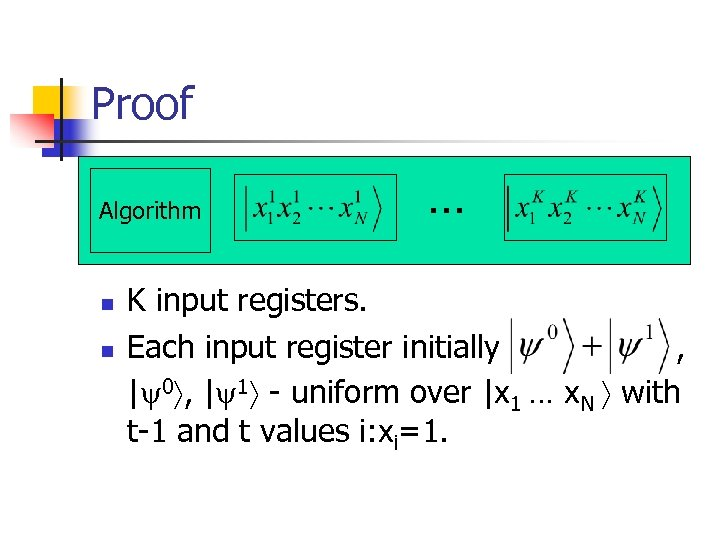Proof Algorithm n n … K input registers. Each input register initially , | 0 , | 1 - uniform over |x 1 … x. N with t-1 and t values i: xi=1.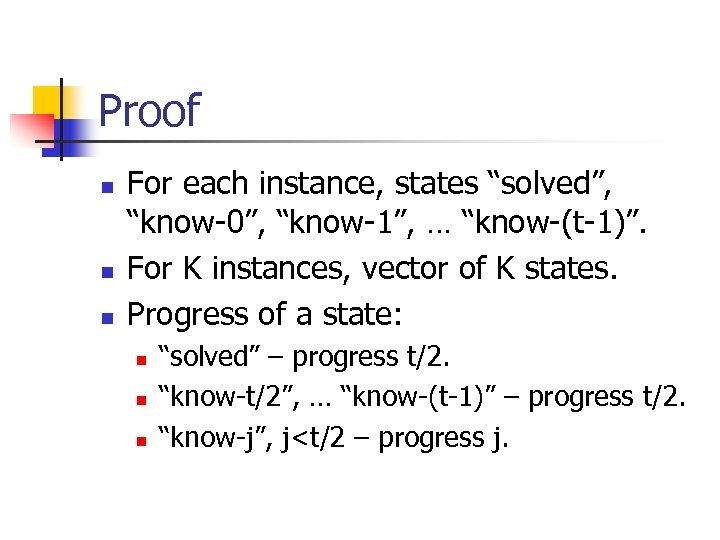Proof n n n For each instance, states “solved”, “know-0”, “know-1”, … “know-(t-1)”. For K instances, vector of K states. Progress of a state: n n n “solved” – progress t/2. “know-t/2”, … “know-(t-1)” – progress t/2. “know-j”, j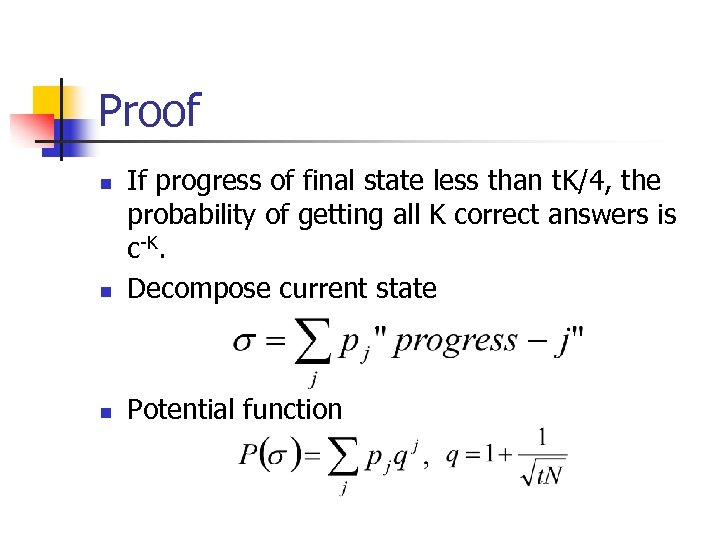Proof n If progress of final state less than t. K/4, the probability of getting all K correct answers is c-K. Decompose current state n Potential function n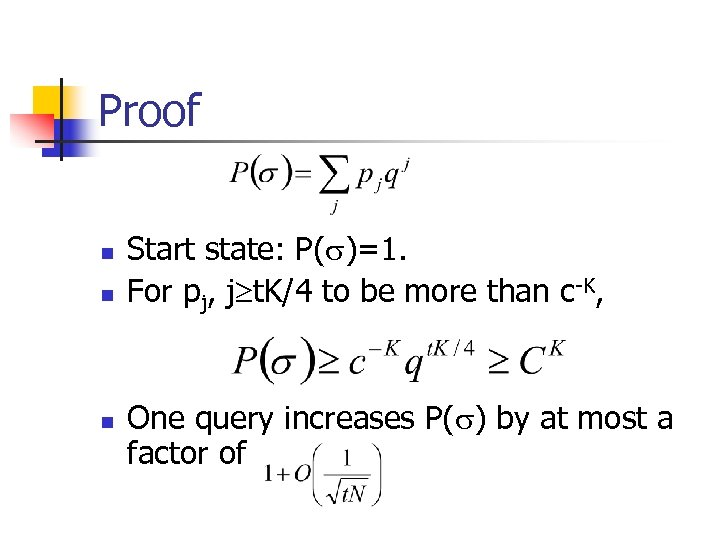Proof n n n Start state: P( )=1. For pj, j t. K/4 to be more than c-K, One query increases P( ) by at most a factor of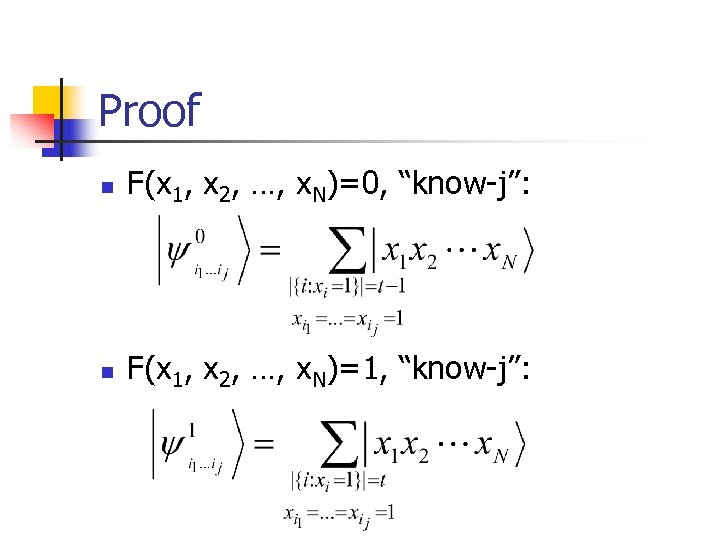Proof n F(x 1, x 2, …, x. N)=0, “know-j”: n F(x 1, x 2, …, x. N)=1, “know-j”: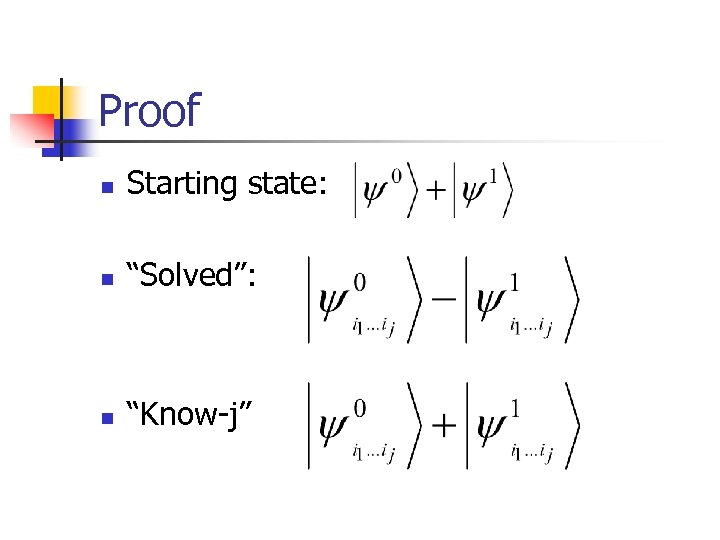Proof n Starting state: n “Solved”: n “Know-j”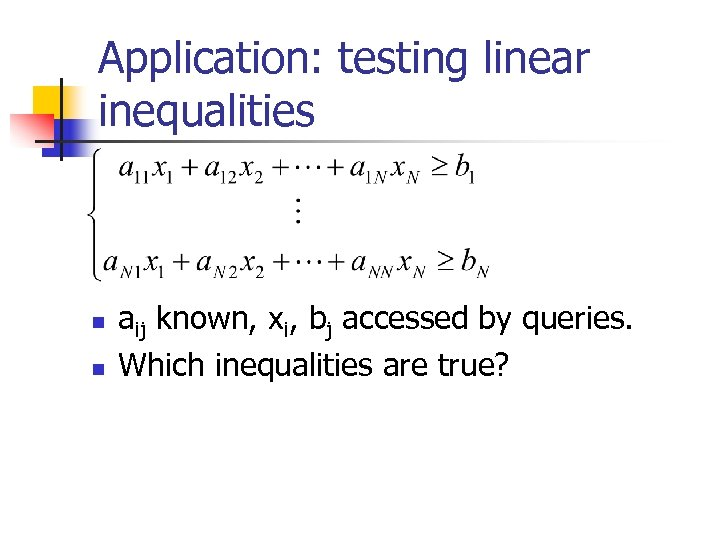Application: testing linear inequalities n n aij known, xi, bj accessed by queries. Which inequalities are true?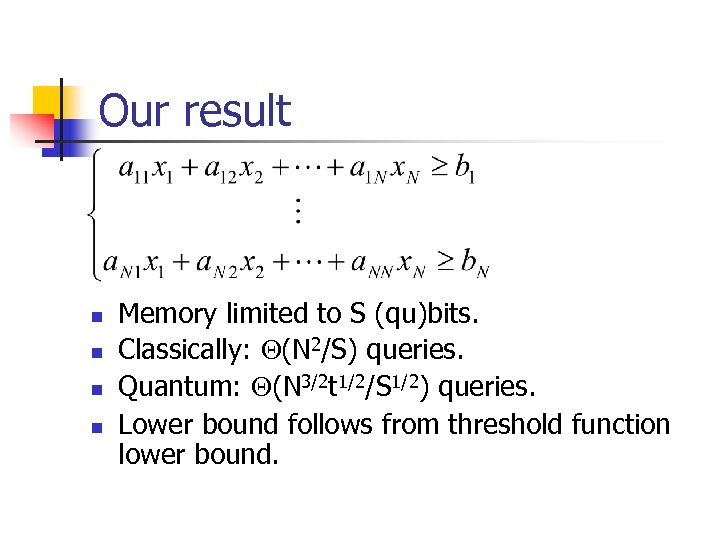Our result n n Memory limited to S (qu)bits. Classically: (N 2/S) queries. Quantum: (N 3/2 t 1/2/S 1/2) queries. Lower bound follows from threshold function lower bound.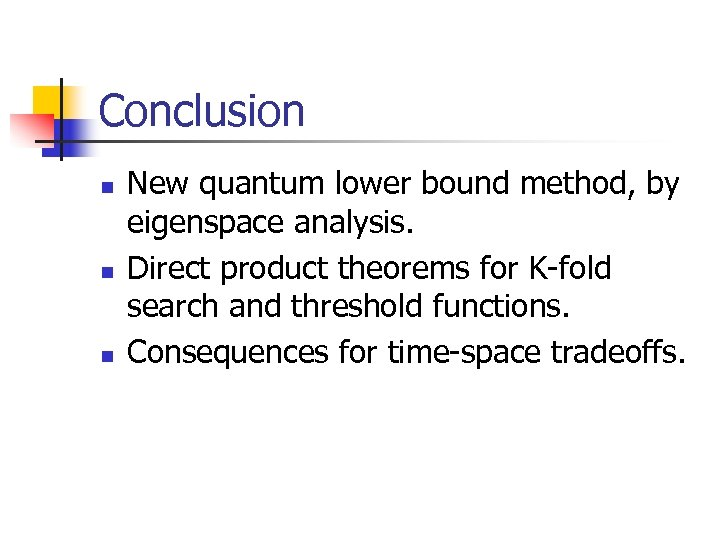Conclusion n New quantum lower bound method, by eigenspace analysis. Direct product theorems for K-fold search and threshold functions. Consequences for time-space tradeoffs.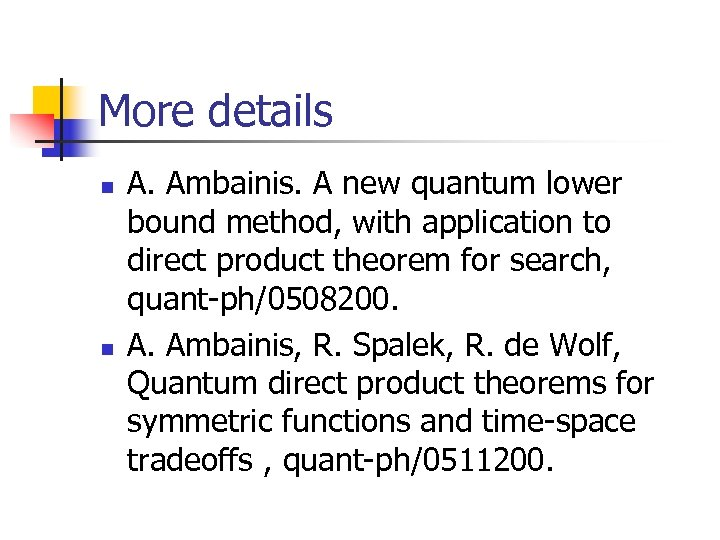More details n n A. Ambainis. A new quantum lower bound method, with application to direct product theorem for search, quant-ph/0508200. A. Ambainis, R. Spalek, R. de Wolf, Quantum direct product theorems for symmetric functions and time-space tradeoffs , quant-ph/0511200.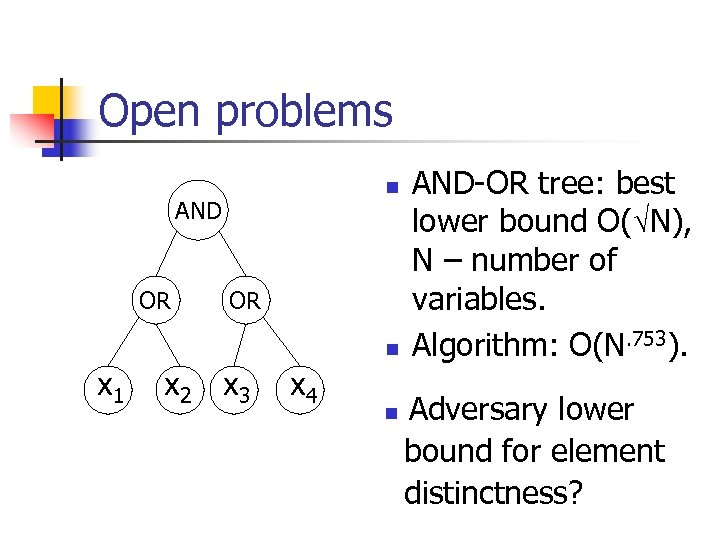Open problems n AND OR OR n x 1 x 2 x 3 x 4 n AND-OR tree: best lower bound O( N), N – number of variables. Algorithm: O(N. 753). Adversary lower bound for element distinctness?